# Arithmetic

Most applications perform arithmetic calculations. The arithmetic operators are summarized in Fig. 3.22. Note the use of various special symbols not used in algebra. The asterisk (*) indicates multiplication, and the percent sign (%) is the remainder operator (called modulus in some languages), which we will discuss shortly. The arithmetic operators in Fig. 3.22 are binary operatorsfor example, the expression f + 7 contains the binary operator + and the two operands f and 7.

Figure 3.22. Arithmetic operators.

C# operation

Arithmetic operator

Algebraic expression

C# expression

+

f + 7

f + 7

Subtraction

p c

p - c

Multiplication

*

b · m

b * m

Division

/

x / y or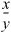or x ÷ y

x / y

Remainder

%

r mod s

r % s

Integer division yields an integer quotientfor example, the expression 7 / 4 evaluates to 1, and the expression 17 / 5 evaluates to 3. Any fractional part in integer division is simply discarded (i.e., truncated)no rounding occurs. C# provides the remainder operator, %, which yields the remainder after division. The expression x % y yields the remainder after x is divided by y. Thus, 7 % 4 yields 3, and 17 % 5 yields 2. This operator is most commonly used with integer operands, but can also be used with floats, doubles, and decimals. In the chapter's exercises and in later chapters, we consider several interesting applications of the remainder operator, such as determining whether one number is a multiple of another.

Arithmetic expressions must be written in straight-line form to facilitate entering applications into the computer. Thus, expressions such as "a divided by b" must be written as a / b, so that all constants, variables and operators appear in a straight line. The following algebraic notation is generally not acceptable to compilers: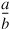Parentheses are used to group terms in C# expressions in the same manner as in algebraic expressions. For example, to multiply a times the quantity b + c, we write

```a * ( b + c )
```

If an expression contains nested parentheses, such as

```( ( a + b ) * c )
```

the expression in the innermost set of parentheses (a + b in this case) is evaluated first.

C# applies the operators in arithmetic expressions in a precise sequence determined by the following rules of operator precedence, which are generally the same as those followed in algebra (Fig. 3.23):

1. Multiplication, division and remainder operations are applied first. If an expression contains several such operations, the operators are applied from left to right. Multiplication, division and remainder operators have the same level of precedence.
2. Addition and subtraction operations are applied next. If an expression contains several such operations, the operators are applied from left to right. Addition and subtraction operators have the same level of precedence.

Figure 3.23. Precedence of arithmetic operators.

Operator(s)

Operation(s)

Order of evaluation (associativity)

Evaluated first

*

/

%

Multiplication

Division

Remainder

If there are several operators of this type, they are evaluated from left to right.

Evaluated next

+

-

Subtraction

If there are several operators of this type, they are evaluated from left to right.

These rules enable C# to apply operators in the correct order. When we say that operators are applied from left to right, we are referring to their associativity. You will see that some operators associate from right to left. Figure 3.23 summarizes these rules of operator precedence. The table will be expanded as additional operators are introduced. A complete precedence chart is included in Appendix A.

Now let us consider several expressions in light of the rules of operator precedence. Each example lists an algebraic expression and its C# equivalent. The following is an example of an arithmetic mean (average) of five terms:

 Algebra: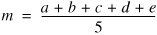C#: m = ( a + b + c + d + e ) / 5;

The parentheses are required because division has higher precedence than addition. The entire quantity ( a + b + c + d + e ) is to be divided by 5. If the parentheses are erroneously omitted, we obtain a + b + c + d + e / 5, which evaluates as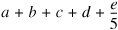The following is an example of the equation of a straight line:

 Algebra: y = mx + b; C#: y = m * x + b;

No parentheses are required. The multiplication operator is applied first because multiplication has a higher precedence than addition. The assignment occurs last because it has a lower precedence than multiplication or addition.

The following example contains remainder (%), multiplication, division, addition and subtraction operations:The circled numbers under the statement indicate the order in which C# applies the operators. The multiplication, remainder and division operations are evaluated first in left-to-right order (i.e., they associate from left to right), because they have higher precedence than addition and subtraction. The addition and subtraction operations are evaluated next. These operations are also applied from left to right.

To develop a better understanding of the rules of operator precedence, consider the evaluation of a second-degree polynomial (y = ax2 + bx + c):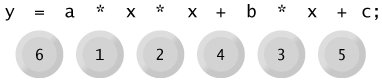The circled numbers indicate the order in which C# applies the operators. The multiplication operations are evaluated first in left-to-right order (i.e., they associate from left to right), because they have higher precedence than addition. The addition operations are evaluated next and are applied from left to right. There is no arithmetic operator for exponentiation in C#, so x2 is represented as x * x. Section 6.4 shows an alternative for performing exponentiation in C#.

Suppose that a, b, c and x in the preceding second-degree polynomial are initialized (given values) as follows: a = 2, b = 3, c = 7 and x = 5. Figure 3.24 illustrates the order in which the operators are applied.

Figure 3.24. Order in which a second-degree polynomial is evaluated.

(This item is displayed on page 102 in the print version)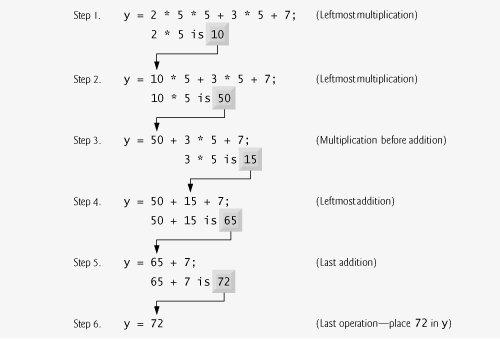As in algebra, it is acceptable to place unnecessary parentheses in an expression to make the expression clearer. These are called redundant parentheses. For example, the preceding assignment statement might be parenthesized to highlight its terms as follows:

```y = ( a * x * x ) + ( b * x ) + c;
```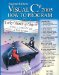Visual C# 2005 How to Program (2nd Edition)
ISBN: 0131525239
EAN: 2147483647
Year: 2004
Pages: 600